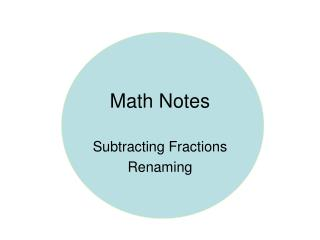DownloadDownload PresentationMath Notes

# Math Notes

Télécharger la présentation## Math Notes

- - - - - - - - - - - - - - - - - - - - - - - - - - - E N D - - - - - - - - - - - - - - - - - - - - - - - - - - -
##### Presentation Transcript

1. Math Notes Subtracting Fractions Renaming

2. Subtracting Fractions • Subtracting mixed numbers is just like subtracting fractions, except that you have to subtract the whole numbers as well as the fractions.

3. 3 Steps • Step One You need to rename before you subtract when the top or first fraction is less than the bottom or second fraction. Example: 4 1 8 - 5 1 8

4. Rename You borrow from the whole number four. • 1 = 3 8 + 1 = 3 9 8 8 8 8

5. Step 2 • Subtract the fractions and the whole numbers. • 3 9 - 1 5 = 2 4 8 8 8

6. Step 3 • Simplify your answer. 2 4 = 2 1 8 2